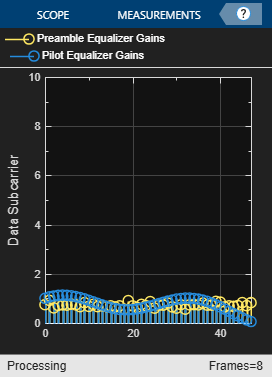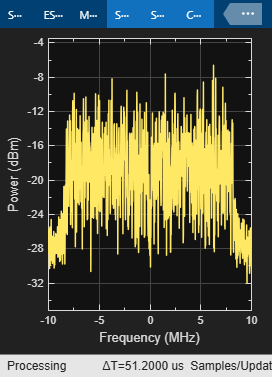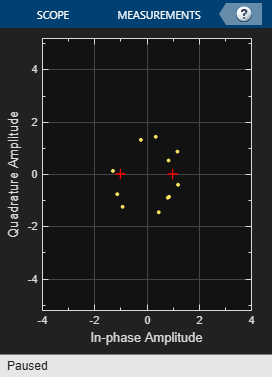# OFDM Synchronization

This example shows a method for digital communication with OFDM synchronization based upon the IEEE® 802.11a™ standard. System objects™ from the Communications Toolbox™ are utilized to provide OFDM modulation and demodulation and help synchronization functionality. In particular, this example illustrates methods to address real-world wireless communication issues like carrier frequency recovery, timing recovery, and frequency domain equalization.

### Implementations

This example describes a MATLAB® implementation of OFDM synchronization, based upon the IEEE 802.11a standard [ 3 ].

### Introduction

The IEEE 802.11a standard describes the transmission of an OFDM modulated signal for information exchange between systems in local and metropolitan area networks. This example utilizes the physical layer outlined by that standard, specifically the preamble symbols and the OFDM grid structure.

The purpose of this example is:

• To model a general OFDM wireless communication system that is able to successfully recover a message, which was corrupted by various simulated channel impairments.

• To illustrate the use of key Communications Toolbox tools for OFDM system design and OFDM symbol synchronization

• To illustrate the performance benefits of MATLAB Coder™

### Initialization

Adjustable transmitter parameters including the payload message in each frame that consists of several OFDM symbols and the number of transmitted frames.

```message = 'Live long and prosper, from the Communications Toolbox Team at MathWorks!'; numFrames = 1e2; % Adjustable channel parameters EbN0dB = 12; % Channel noise level (dB) frequencyOffset = 1e4; % Frequency offset (Hz) phaseOffset = 15; % Phase offset (Degrees) delay = 80; % Initial sample offset for entire data stream (samples) % Display recovered messages displayRecoveredMsg = false; % Enable scope visualizations useScopes = true; % Check for MATLAB Coder license useCodegen = checkCodegenLicense; if useCodegen fprintf(['--MATLAB Coder license found. ',... 'Transmitter and receiver functions will be compiled for ',... 'additional simulation acceleration.--\n']); end % By default the transmitter and receiver functions will be recompiled % between every run, which is not always necessary. To disable receiver % compilation, change "compileIt" to false. compileIt = useCodegen; ```
```--MATLAB Coder license found. Transmitter and receiver functions will be compiled for additional simulation acceleration.-- ```

### Code Architecture for the System

This example models a digital communication system based upon the IEEE 802.11a standard [ 3 ]. The system is broken down into four functions: generateOFDMSignal, applyOFDMChannel, receiveOFDMSignal, and calculateOFDMBER.

1) generateOFDMSignal: set up and call an OFDMTransmitter System object. The object converts the payload message into a bit stream which is first PSK modulated, then OFDM modulated, and finally prepended by preamble OFDM symbols to form an individual frame. The transmitter repeats this frame `numFrames` times.

2) applyOFDMChannel: models the channel with carrier offset, timing offset, and additive white Gaussian noise (AWGN).

3) receiveOFDMSignal: set up and call an OFDMReceiver System object. The object models a series of components at the receiver, including timing recovery, carrier frequency recovery, channel equalization, and demodulation. The object can also be configured to show multiple scopes to visualize the receiver processing. The output of the OFDMReceiver object is the decoded bit stream from those detected frames.

4) calculateOFDMBER: calculate the system frame error rate (FER) and bit error rate (BER) based on the original payload message in each frame and the bit output from the OFDMReceiver System object.

### Description of the Individual Components and Algorithms

Transmitter

The OFDMTransmitter System object generates an OFDM signal based upon the IEEE 802.11a standard with a supplied ASCII payload. Each transmission frame is made up of several OFDM symbols, including preamble and data symbols. Identical frames are repeated by the transmitter based on the value supplied. Frames are padded to fill the OFDM grid when necessary.

Channel

This component simulates the effects of over-the-air transmission. It degrades the transmitted signal with both phase and frequency offset, a delay to mimic channel delay between transmitter and receiver, and AWGN. The noise level of the AWGN is given in dB.

This OFDMReceiver System object recovers the original transmitted payload message. It is divided into four primary operations in this order:

1) Timing Recovery: This component is responsible for determining the sample location of the start of a given frame. More specifically, it utilizes a known preamble sequence in the received frame found through a cross-correlation. The cross-correlated data will contain a specific peak arrangement/spacing which allows for identification. The preamble itself is designed to produce this specific shape in the time domain. This identification method is based upon [ 1 ]. The locatePreamble method of the object, which is responsible for this operation, uses a normalized minimum peak height, and a minimum number of required peaks to provide a possible preamble match.

2) Carrier Frequency Recovery: Frequency estimation is accomplished by calculating the phase difference in the time domain between halves of the long portion of the 802.11a preamble. This phase difference Phi is then converted to a frequency offset. This is a common technique originally published by Schmidl and Cox [ 2 ]. This implementation of the phase measurement assumes that the true offset is within pi, or one frequency bin of the FFT. In the case of 802.11a a bin is 312.5kHz wide.

3) Frequency Domain Equalization: Since the frequency estimate can be inaccurate, additional phase rotation will exist at the subcarrier level of the OFDM symbol. As well as phase rotations, channel fading will also affect the received signal. Both of these impairments are corrected by a frequency domain equalizer. The equalizer has two stages, utilizing both preamble and pilot data. First, the received payload is equalized through the use of taps generated from the received long preamble samples. Then the pilot subcarriers are extracted, and interpolated in frequency to provide a full channel estimate. The payload is next equalized using these pilot estimates.

4) Data Decoder: Finally the OFDM subcarriers are demodulated and then, PSK demodulated into bits, from which the original payload message can be recovered.

BER Calculation

This component calculates the system FER and BER based on the original payload message and the decoded bit stream from the detected frames at the receiver. The undetected frames are not counted in the calculation.

Display of Recovered Message

The recovered message at the receiver is displayed for each detected frame. Since the original message length is not sent to the receiver, the padded bits in each frame are also recovered into characters and displayed. So you may see up to 7 meaningless characters at the end of each recovered message.

Scopes

• constellation diagrams showing the received signal before and after frequency domain equalization

• vector plot of the equalizer taps used for a given frame

• a spectrum analyzer displaying detected frames of data

• a time plot displaying the start of detected frames

• a time plot displaying the frequency estimate of the transmitter's carrier offset for detected frames

### OFDM Synchronization Test Overview

A large data vector is regenerated for a given EbN0 value by the generateOFDMSignal function. This data is then passed through the applyOFDMChannel function which introduces several common channel impairments. Finally the data is passed to the receiver for recovery. The receiveOFDMSignal function operates by processing data on a frame-by-frame basis. This processing mechanism is self-contained for performance benefits when using code generation and for code simplicity. This script by default generates code for the transmitter and receiver functions; this is accomplished by using the codegen command provided by the MATLAB Coder product. The codegen command translates MATLAB functions to a C++ static or dynamic library, executable, or to a MEX file, producing a code for accelerated execution. The generated C code runs several times faster than the original MATLAB code.

During operation, the receiver will display a series of plots illustrating certain synchronization results and effects on the signal.

```% Compile transmitter with MATLAB Coder if compileIt codegen generateOFDMSignal -args {coder.Constant(message), coder.Constant(numFrames)} end % Generate transmission signal if useCodegen [txSig, frameLen] = generateOFDMSignal_mex(message, numFrames); else [txSig, frameLen] = generateOFDMSignal(message, numFrames); end % Pass signal through channel rxSig = applyOFDMChannel(txSig, EbN0dB, delay, frequencyOffset, phaseOffset); % Compile receiver with MATLAB Coder if compileIt codegen receiveOFDMSignal -args {rxSig, coder.Constant(frameLen), coder.Constant(displayRecoveredMsg), coder.Constant(useScopes)} end % Recover signal if useCodegen [decMsgInBits, numFramesDetected] = receiveOFDMSignal_mex(rxSig, frameLen, displayRecoveredMsg, useScopes); else [decMsgInBits, numFramesDetected] = receiveOFDMSignal(rxSig, frameLen, displayRecoveredMsg, useScopes); end % Calculate average BER [FER, BER] = calculateOFDMBER(message, decMsgInBits, numFramesDetected); fprintf('\nAt EbNo = %5.2fdB, %d frames detected among the %d transmitted frames with FER = %f and BER = %f\n', ... EbN0dB, numFramesDetected, numFrames, FER, BER); ```
```Code generation successful. Code generation successful. At EbNo = 12.00dB, 100 frames detected among the 100 transmitted frames with FER = 0.010000 and BER = 0.000098 ```### Summary

This example utilizes several MATLAB System objects to simulate digital communication with OFDM over an AWGN channel. It shows how to model several parts of the OFDM system such as modulation, frequency estimation, timing recovery, and equalization. The simulation also displays information about the operation of the synchronization algorithms through a series of plots. This example also utilizes code generation, allowing the simulation to run several times faster than the original MATLAB code.

### Appendix

The following System objects are used in this example:

The following helper functions are used in this example:

### References

1. Minn, H.; Zeng, M.; Bhargava, V.K., "On timing offset estimation for OFDM systems," Communications Letters, IEEE , vol.4, no.7, pp.242,244, July 2000

2. Schmidl, T.M.; Cox, D.C., "Robust frequency and timing synchronization for OFDM," Communications, IEEE Transactions on , vol.45, no.12, pp.1613,1621, Dec 1997

3. IEEE Std 802.11a, "Part 11: Wireless LAN Medium Access Control (MAC) and Physical Layer (PHY) Specifications," 1999.